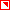# Thermal Ellipsoids from Experiment and Theory

## Experimental

Anisotropic displacement parameters (= thermal ellipsoids or just "ellipsoids" or "ORTEPs") serve to model the thermal motions of atoms when refining X-ray or neutron diffraction data.We all know that atoms in crystal structures move around their equilibrium positions at any temperature. This motion lowers the scattering factors of the atoms and must be considered during a crystal-structure refinement. This is typically done with the help of a Gaussian function and by introducing a vibration amplitude in the form of a symmetric tensor ├ó┬?┬? the matrix of the anisotropic displacement parameters. The latter is what we calculate in silico. This matrix can be easily transformed into thermal ellipsoids ├ó┬?┬? the volume in which the centers of gravity of the atoms are found with a certain probability.

If you're an experimental crystallographer you have very likely come across those "Uani" tags in refinement procedures, but if you're new to the field we may recommend an excellent first introduction at the following site:
http://www.crystallographiccourseware.com/ThermalParameters/ThermalParameters.html.

For a more advanced (and mathematically rigid) background, we may recommendhttp://ww1.iucr.org/comm/cnom/adp/finrep/ or, of course, the crystallography textbook of your choice.

## And Why from Theory?

Because thermal ellipsoids are experimental observables, they can also be calculated from quantum theory. Although observables are most easily observed (and not computed), there are several cases where the experimental approach faces trouble. For example, hydrogen ADPs (or the atoms themselves!) may be invisible in X-ray diffraction, but those atoms are those which move the most. Neutron diffraction can alleviate this issue, but is an expensive technique and requires very large crystals. As alluded to already, quantum theory can help out in many of these cases, at least in principle.

To illustrate the good agreement between the result from X-ray diffraction and quantum theory at 100 K, we show the following visual comparison of the thermal ellipsoids for pentachloropyridine:Adapted fromCrystEngComm 2015, 17, 7414. The article is licensed under a Creative Commons Attribution-NonCommercial 3.0 Unported Licence.

## How It's Done

There are different ways to calculate ORTEPs:
• Cluster models combined with multi-level simulations (ONIOM model): This is a clever trick which splits the quantum-chemical calculation into a high- and a low-level part so that the ADPs of the atoms can be calculated efficiently in their chemical environment ├ó┬?┬? the crystal ├ó┬?┬? approximated by a cluster. For more information about this approach, see, for example,Acta Cryst. A 2012, 68, 110.

• Molecular-dynamics (MD) simulations: An MD trajectory contains information about how the atoms in a structure move over time, and by collecting this information one can extract ADPs. This is particularly useful in cases of room- and high-temperature settings. For more information about this approach, see, for example,Chem. Phys. Lett. 2007, 448, 61 andAppl. Crystallogr., 2013, 46, 656 .

• Periodic lattice-dynamics calculations: This approach originated from the physics communities and, accordingly, the first applications were concerned with semiconductors and metal carbides, but it can be used for organic/molecular crystals just as well, as it has been shown by us and others (seeBasic References). More information about periodic lattice dynamics in general can be found inWallace, Duane C., Thermodynamics of Crystals. New York: Wiley, 1972.

The latter is what this page is about.

## Theoretical Background

The ADP matrix, here in the Cartesian coordinate system (= Ucart matrix ), serves to describe the thermal ellipsoids of an atom j. It can be calculated with the following formula:withIn this formula, N is the number of unit cells, mj is the mass of the atom j, ├Å┬?├?┬Į(q) is the frequency mode of the phonon band ├?┬Į, e├?┬Į(j,q) is the polarization vector of atom j, the phonon band ├?┬Į and q is the wave vector. All parameters are accessible by harmonic phonon calculations.

The formula is taken from
http://atztogo.github.io/phonopy/thermal-displacement.html#mean-square-displacement-matrix (accessed on 14th, April 2016).
last modified: 2016-09-26